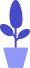# Python if else conditional statement

Estimated reading: 6 minutes 76 views

Conditional statements are used to determine whether a given condition satisfies the requirements, and the next action is determined according to the result of the determination. Conditional statements are a control structure because they allow you to control which part of the code is executed when, based on specific conditions you define.

Conditional statements are one of the most important concepts in Python. In Python, a conditional statement begins with the if keyword, followed by a condition and a colon `：`, and the line of code after the colon is placed in a code block. If the condition is met, the instruction of the code block is executed. The format is as follows, and the code is as follows.

``````if 3>2 :
print("Three is greater than two")``````

The output is shown in Figure 1.figure 1
In this conditional statement, since the result of 3>2 is True, the string “Three is greater than two” is printed.

## if statement

The if statement is used in programming languages ​​to determine whether a given condition is satisfied, and to decide which operations to perform according to the result of the determination – if the condition is True, execute the code block, if the condition is False, skip without executing the following statement.

In Python, an if statement contains the following parts

• if keyword;
• condition;
• colon;
• An indented block of code (i.e. the body), starting on the next line.

An if statement has two main parts: the condition and the body. The condition should be a boolean. The body is one or more lines of code. If the condition is True, the code can be executed.

For example, we assign the content entered by the user to the variable name, and then judge whether the content of the assignment is equal to “Johnson”, if it is equal, print “Hello my son.”, the code is as follows.

``````name=input("Please input your name：")
if name=="Robort":
print ("Hello my son.")``````

It can be seen that when the input content is “Johnson” and the condition name = “Johnson” is met, “Hello my son.” will be printed, and if other content is entered, because the condition is not met, nothing will be printed. ,as shown in picture 2.

## else statement

We learned earlier that an if statement executes a block of code only if the condition is True. If the condition is False and we still want to do something, we need to use an if…else statement. The if…else statement looks similar to the if statement, except that it has two code blocks. The keyword else is placed between two code blocks. In an if…else statement, the else clause will only execute when the if statement condition is False.

There is no condition in the else statement, in the code, the else statement contains the following parts:

• else keyword;
• colon;
• Indented code blocks starting on the next line

Let’s go back to the previous example, this time add an else statement, if the name is not Johnson, it will say “Hello my friend.”, the code is as follows.

``````name=input("Please input your name：")
if name=="Johnson":
print ("Hello my son. ")
else:
print ("Hello my friend.")``````

This time, if instead of “Johnson” but “Alex”, the program prints “Hello my friend.”, as shown in Figure 3.

Figure 3

## elif statement

We often need to check a series of conditions. When one of the conditions is True, we will do the corresponding things. At this time, we need to create a series of if…else statements.

In this case, start with a regular if statement, and after the body’s code block, enter the keyword elif, followed by another condition and another block of statements. We can keep doing this until all conditions are executed, and there is no limit to the number of conditions. If none of the conditions are true, the last else part is executed.

The elif statement contains the following parts:

• elif keyword;
• condition;
• colon;
• An indented block of code starting on the next line.

Let’s continue with the example just now:

• If the user entered “Johnson”, the string to be printed is “Hello my son.”;
• If the user enters “Judy”, the string to print is “Hello my daughter.”;
• If you enter “Aric”, the string to be printed is “Hello my friend”;
• If it is “John”, then say hello to yourself “Hello to myself.”;
• If none of the above, the string to be printed becomes “Hello others.”.

We can have as many elif clauses as we want, the code is as follows.

``````name=input("Please input your name：")
if name=="Johnson":
print("Hello my son.")
elif name=="Judy":
print("Hello my daughter.")
elif name=="Aric":
print("Hello my friend.")
elif name=="John":
print("Hello to myself.")
else:
print("Hello others.")``````

We can interpret these elif statements as follows

• If the first condition is True, execute the first code block;
• If the second condition is True, execute the second code block;
• If the 3rd condition is True, execute the 3rd code block;
• ……
• If the above conditions are all False, the else part is executed.

When using such a string of if…else statements with an elif part, we can ensure that only one block of code will be executed. When a condition is found to be True, the corresponding code block will be executed without verifying other conditions. If none of the conditions are True, the else block is executed.

One more thing to note: the else at the end is optional. However, without this else, when all conditions are not true, the contents of the if…else statement block will not be executed, the code is as follows.

``````name=input("Please input your name：")
if name=="Johnson":
print("Hello my son.")
elif name=="Judy":
print("Hello my daughter.")
elif name=="Aric":
print("Hello my friend.")
elif name=="John":
print("Hello to myself.")``````

The code above omits the final else part, and when “Peter” is entered, nothing will be printed because that’s not the person you want to greet, as shown in Figure 4.